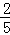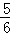首页 / 小学数学 / 试卷选题

# 北京版六年级上第一章分数乘法同步练习

 A．B．C．D．• 题型：未知
• 难度：未知

A．真分数     B．假分数     C．整数

• 题型：未知
• 难度：未知

20××20相比较，它们的（　　）

 A．意义和计算结果都相同 B．意义相同，计算结果不同 C．意义不同，计算结果相同 D．意义和计算结果都不相同

• 题型：未知
• 难度：未知

A．女生人数×=男生人数  B．男生人数×=女生人数

• 题型：未知
• 难度：未知

m是非0的自然数，下列算式中得数最大的是（　　）

 A．m﹣B．m÷C．m×D．m+• 题型：未知
• 难度：未知

5吨的的重量（　　）5个吨的重量．
A．＞     B．＜     C．=

• 题型：未知
• 难度：未知

50的是（　　）
A．100   B．50C．25

• 题型：未知
• 难度：未知

1米的是（　　）分米．
A．10     B．1       C．• 题型：未知
• 难度：未知

3个的和是（　　）
A．1      B．C．3

• 题型：未知
• 难度：未知

A．大于 B．小于 C．等于

• 题型：未知
• 难度：未知

1小时的是（　　）

 A．18分钟 B．24分钟 C．30分钟 D．36分钟

• 题型：未知
• 难度：未知

• 题型：未知
• 难度：未知

45的，一个数的是50，这个数是

• 题型：未知
• 难度：未知的意义是

• 题型：未知
• 难度：未知×4 结果相等，所以意义也相同．

• 题型：未知
• 难度：未知

• 题型：未知
• 难度：未知

• 题型：未知
• 难度：未知

• 题型：未知
• 难度：未知

• 题型：未知
• 难度：未知

4分米的和5分米的相等．　　　　　　．（判断对错）

• 题型：未知
• 难度：未知

• 题型：未知
• 难度：未知×24= 2.4÷0.04= 125×8= 6÷=﹣= （+）×18=

• 题型：未知
• 难度：未知×==
10﹣=+=
500﹣399=
12×（+）=

• 题型：未知
• 难度：未知

 10×85%= 8.1÷0.03= 3﹣= 200×3.6= 217﹣18= 134﹣18= 1.5×4= 7.45+8.55=

• 题型：未知
• 难度：未知• 题型：未知
• 难度：未知